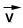## TyroCity

Physics XI Notes for Physics Notes

Posted on • Updated on

# Resolution Of Vector

Definition

The process of splitting a vector into various parts or components is called resolution of vector.
These parts of a vector may act in different directions and are called “components of vector”.
We can resolve a vector into a number of components. Generally there are three components of vector viz.

• Component along X-axis called x-component
• Component along Y-axis called Y-component
• Component along Z-axis called Z-component

Here we will discuss only two components x-component & Y-component which are perpendicular to each other. These components are called rectangular components of vector.

Consider a vectoracting at a point making an angle q with positive X-axis. Vectoris represented by a line OA. From point A draw a perpendicular AB on X-axis. Suppose OB and BA
represents two vectors. Vector OA is parallel to X-axis and vector BA is parallel to Y-axis. Magnitude
of these vectors are Vx and Vy respectively. By the method of head to tail we notice that the sum of these vectors is equal to vectorThus, Vx and Vy are the rectangular components of vectorMagnitude of horizontal component

Consider right-angled triangle DOABMagnitude of vertical component